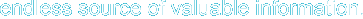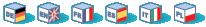# Introduction

PHP supports libcurl, a library created by Daniel Stenberg, that allows you to connect and communicate to many different types of servers with many different types of protocols. libcurl currently supports the http, https, ftp, gopher, telnet, dict, file, and ldap protocols. libcurl also supports HTTPS certificates, HTTP POST, HTTP PUT, FTP uploading (this can also be done with PHP's ftp extension), HTTP form based upload, proxies, cookies, and user+password authentication.

These functions have been added in PHP 4.0.2.

# Requirements

In order to use the CURL functions you need to install the CURL package. PHP requires that you use CURL 7.0.2-beta or higher. In PHP 4.2.3, you will need CURL version 7.9.0 or higher. From PHP 4.3.0, you will need a CURL version that's 7.9.8 or higher. PHP 5.0.0 requires a CURL version 7.10.5 or greater.

# Installation

To use PHP's CURL support you must also compile PHP --with-curl[=DIR] where DIR is the location of the directory containing the lib and include directories. In the "include" directory there should be a folder named "curl" which should contain the easy.h and curl.h files. There should be a file named libcurl.a located in the "lib" directory. Beginning with PHP 4.3.0 you can configure PHP to use CURL for URL streams --with-curlwrappers.

Note to Win32 Users: In order to enable this module on a Windows environment, you must copy libeay32.dll and ssleay32.dll from the DLL folder of the PHP/Win32 binary package to the SYSTEM folder of your Windows machine. (Ex: C:\WINNT\SYSTEM32 or C:\WINDOWS\SYSTEM)

# Predefined Constants

The constants below are defined by this extension, and will only be available when the extension has either been compiled into PHP or dynamically loaded at runtime.

CURLOPT_AUTOREFERER (integer)

Available since PHP 5.1.0

Available since PHP 5.1.0

CURLOPT_DNS_USE_GLOBAL_CACHE (integer)

CURLOPT_DNS_CACHE_TIMEOUT (integer)

CURLOPT_FTPSSLAUTH (integer)

Available since PHP 5.1.0

CURLOPT_PORT (integer)

CURLOPT_FILE (integer)

CURLOPT_INFILE (integer)

CURLOPT_INFILESIZE (integer)

CURLOPT_URL (integer)

CURLOPT_PROXY (integer)

CURLOPT_VERBOSE (integer)

CURLOPT_NOPROGRESS (integer)

CURLOPT_NOBODY (integer)

CURLOPT_FAILONERROR (integer)

CURLOPT_POST (integer)

CURLOPT_FTPLISTONLY (integer)

CURLOPT_FTPAPPEND (integer)

CURLOPT_NETRC (integer)

CURLOPT_FOLLOWLOCATION (integer)

CURLOPT_FTPASCII (integer)

CURLOPT_PUT (integer)

CURLOPT_MUTE (integer)

CURLOPT_USERPWD (integer)

CURLOPT_PROXYUSERPWD (integer)

CURLOPT_RANGE (integer)

CURLOPT_TIMEOUT (integer)

CURLOPT_POSTFIELDS (integer)

CURLOPT_REFERER (integer)

CURLOPT_USERAGENT (integer)

CURLOPT_FTPPORT (integer)

CURLOPT_FTP_USE_EPSV (integer)

CURLOPT_LOW_SPEED_LIMIT (integer)

CURLOPT_LOW_SPEED_TIME (integer)

CURLOPT_RESUME_FROM (integer)

CURLOPT_SSLCERT (integer)

CURLOPT_SSLCERTPASSWD (integer)

CURLOPT_SSL_VERIFYHOST (integer)

CURLOPT_SSLVERSION (integer)

CURLOPT_TIMECONDITION (integer)

CURLOPT_TIMEVALUE (integer)

CURLOPT_CUSTOMREQUEST (integer)

CURLOPT_STDERR (integer)

CURLOPT_TRANSFERTEXT (integer)

CURLOPT_RETURNTRANSFER (integer)

CURLOPT_QUOTE (integer)

CURLOPT_POSTQUOTE (integer)

CURLOPT_INTERFACE (integer)

CURLOPT_KRB4LEVEL (integer)

CURLOPT_HTTPPROXYTUNNEL (integer)

CURLOPT_FILETIME (integer)

CURLOPT_WRITEFUNCTION (integer)

CURLOPT_PASSWDFUNCTION (integer)

CURLOPT_MAXREDIRS (integer)

CURLOPT_MAXCONNECTS (integer)

CURLOPT_CLOSEPOLICY (integer)

CURLOPT_FRESH_CONNECT (integer)

CURLOPT_FORBID_REUSE (integer)

CURLOPT_RANDOM_FILE (integer)

CURLOPT_EGDSOCKET (integer)

CURLOPT_CONNECTTIMEOUT (integer)

CURLOPT_SSL_VERIFYPEER (integer)

CURLOPT_CAINFO (integer)

CURLOPT_CAPATH (integer)

CURLOPT_SSL_CIPHER_LIST (integer)

CURLOPT_BINARYTRANSFER (integer)

CURLOPT_NOSIGNAL (integer)

CURLOPT_PROXYTYPE (integer)

CURLOPT_BUFFERSIZE (integer)

CURLOPT_HTTPGET (integer)

CURLOPT_HTTP_VERSION (integer)

CURLOPT_SSLKEY (integer)

CURLOPT_SSLKEYTYPE (integer)

CURLOPT_SSLKEYPASSWD (integer)

CURLOPT_SSLENGINE (integer)

CURLOPT_SSLENGINE_DEFAULT (integer)

CURLOPT_SSLCERTTYPE (integer)

CURLOPT_CRLF (integer)

CURLOPT_ENCODING (integer)

CURLOPT_PROXYPORT (integer)

CURLOPT_UNRESTRICTED_AUTH (integer)

CURLOPT_FTP_USE_EPRT (integer)

CURLOPT_HTTP200ALIASES (integer)

CURLOPT_HTTPAUTH (integer)

CURLAUTH_BASIC (integer)

CURLAUTH_DIGEST (integer)

CURLAUTH_GSSNEGOTIATE (integer)

CURLAUTH_NTLM (integer)

CURLAUTH_ANY (integer)

CURLAUTH_ANYSAFE (integer)

CURLOPT_PROXYAUTH (integer)

CURLCLOSEPOLICY_LEAST_RECENTLY_USED (integer)

CURLCLOSEPOLICY_LEAST_TRAFFIC (integer)

CURLCLOSEPOLICY_SLOWEST (integer)

CURLCLOSEPOLICY_CALLBACK (integer)

CURLCLOSEPOLICY_OLDEST (integer)

CURLINFO_EFFECTIVE_URL (integer)

CURLINFO_HTTP_CODE (integer)

CURLINFO_REQUEST_SIZE (integer)

CURLINFO_TOTAL_TIME (integer)

CURLINFO_NAMELOOKUP_TIME (integer)

CURLINFO_CONNECT_TIME (integer)

CURLINFO_PRETRANSFER_TIME (integer)

CURLINFO_FILETIME (integer)

CURLINFO_SSL_VERIFYRESULT (integer)

CURLINFO_STARTTRANSFER_TIME (integer)

CURLINFO_CONTENT_TYPE (integer)

CURLINFO_REDIRECT_TIME (integer)

CURLINFO_REDIRECT_COUNT (integer)

CURL_VERSION_IPV6 (integer)

CURL_VERSION_KERBEROS4 (integer)

CURL_VERSION_SSL (integer)

CURL_VERSION_LIBZ (integer)

CURLVERSION_NOW (integer)

CURLE_OK (integer)

CURLE_UNSUPPORTED_PROTOCOL (integer)

CURLE_FAILED_INIT (integer)

CURLE_URL_MALFORMAT (integer)

CURLE_URL_MALFORMAT_USER (integer)

CURLE_COULDNT_RESOLVE_PROXY (integer)

CURLE_COULDNT_RESOLVE_HOST (integer)

CURLE_COULDNT_CONNECT (integer)

CURLE_FTP_ACCESS_DENIED (integer)

CURLE_FTP_WEIRD_227_FORMAT (integer)

CURLE_FTP_CANT_GET_HOST (integer)

CURLE_FTP_CANT_RECONNECT (integer)

CURLE_FTP_COULDNT_SET_BINARY (integer)

CURLE_PARTIAL_FILE (integer)

CURLE_FTP_COULDNT_RETR_FILE (integer)

CURLE_FTP_WRITE_ERROR (integer)

CURLE_FTP_QUOTE_ERROR (integer)

CURLE_HTTP_NOT_FOUND (integer)

CURLE_WRITE_ERROR (integer)

CURLE_MALFORMAT_USER (integer)

CURLE_FTP_COULDNT_STOR_FILE (integer)

CURLE_OUT_OF_MEMORY (integer)

CURLE_OPERATION_TIMEOUTED (integer)

CURLE_FTP_COULDNT_SET_ASCII (integer)

CURLE_FTP_PORT_FAILED (integer)

CURLE_FTP_COULDNT_USE_REST (integer)

CURLE_FTP_COULDNT_GET_SIZE (integer)

CURLE_HTTP_RANGE_ERROR (integer)

CURLE_HTTP_POST_ERROR (integer)

CURLE_SSL_CONNECT_ERROR (integer)

CURLE_LDAP_CANNOT_BIND (integer)

CURLE_LDAP_SEARCH_FAILED (integer)

CURLE_LIBRARY_NOT_FOUND (integer)

CURLE_FUNCTION_NOT_FOUND (integer)

CURLE_ABORTED_BY_CALLBACK (integer)

CURLE_HTTP_PORT_FAILED (integer)

CURLE_TOO_MANY_REDIRECTS (integer)

CURLE_UNKNOWN_TELNET_OPTION (integer)

CURLE_TELNET_OPTION_SYNTAX (integer)

CURLE_OBSOLETE (integer)

CURLE_SSL_PEER_CERTIFICATE (integer)

CURLE_GOT_NOTHING (integer)

CURLE_SSL_ENGINE_NOTFOUND (integer)

CURLE_SSL_ENGINE_SETFAILED (integer)

CURLE_SEND_ERROR (integer)

CURLE_RECV_ERROR (integer)

CURLE_SHARE_IN_USE (integer)

CURLE_SSL_CERTPROBLEM (integer)

CURLE_SSL_CIPHER (integer)

CURLE_SSL_CACERT (integer)

CURLE_LDAP_INVALID_URL (integer)

CURLE_FILESIZE_EXCEEDED (integer)

CURLE_FTP_SSL_FAILED (integer)

CURLFTPAUTH_DEFAULT (integer)

Available since PHP 5.1.0

CURLFTPAUTH_SSL (integer)

Available since PHP 5.1.0

CURLFTPAUTH_TLS (integer)

Available since PHP 5.1.0

CURLPROXY_HTTP (integer)

CURLPROXY_SOCKS5 (integer)

CURL_NETRC_OPTIONAL (integer)

CURL_NETRC_IGNORED (integer)

CURL_NETRC_REQUIRED (integer)

CURL_HTTP_VERSION_NONE (integer)

CURL_HTTP_VERSION_1_0 (integer)

CURL_HTTP_VERSION_1_1 (integer)

CURLM_CALL_MULTI_PERFORM (integer)

CURLM_OK (integer)

CURLM_OUT_OF_MEMORY (integer)

CURLM_INTERNAL_ERROR (integer)

CURLMSG_DONE (integer)

# Examples

Once you've compiled PHP with CURL support, you can begin using the CURL functions. The basic idea behind the CURL functions is that you initialize a CURL session using the curl_init(), then you can set all your options for the transfer via the curl_setopt(), then you can execute the session with the curl_exec() and then you finish off your session using the curl_close(). Here is an example that uses the CURL functions to fetch the example.com homepage into a file:

Example 1. Using PHP's CURL module to fetch the example.com homepage

 ``` ```

curl_close -- Close a CURL session
curl_copy_handle --  Copy a cURL handle along with all of its preferences
curl_errno -- Return the last error number
curl_error --  Return a string containing the last error for the current session
curl_exec -- Perform a CURL session
curl_getinfo --  Get information regarding a specific transfer
curl_init -- Initialize a CURL session
curl_multi_add_handle --  Add a normal cURL handle to a cURL multi handle
curl_multi_close --  Close a set of cURL handles
curl_multi_exec --  Run the sub-connections of the current cURL handle
curl_multi_getcontent --  Return the content of a cURL handle if CURLOPT_RETURNTRANSFER is set